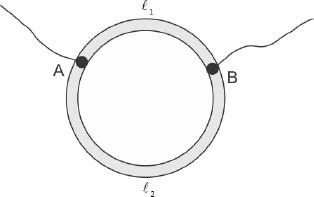#### A ring is made of a wire having a resistance R0 = 12. Find the points A and B as shown in the figure, at which a current carrying conductor should be connected so that the resistance R of the sub circuit between these points is equal toOption 1)Option 2)Option 3)Option 4)net n be resistance per unit length of the wire

Upper PortionLower PortionEquivalent resistance between A and B is-------------------------(i)12=-----------------------------------(ii)oror=Let y ==>=>or 2Option 1)This option is incorrect

Option 2)This option is incorrect

Option 3)This option is incorrect

Option 4)This option is correct